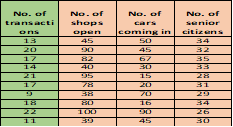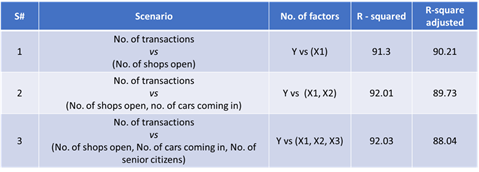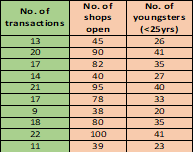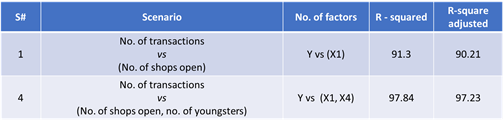• 0

# R Squared

R-Squared

R-Squared is also known as the Coefficient of Determination and is an output from regression analysis. It represents the percentage of response variable variation that is explained by its relationship with one or more predictor variables. In general, the higher the R-squared value, the better the model fits your data. It is always between 0 and 100%.

R-Squared Adjusted is a modified version of R-Squared value. In addition to explaining the percentage of response variable variation that is explained by its relationship with one or more predictor variables, it also takes into account the number of predictor variables. It increases if an additional predictor improves the model more than what is expected by chance. R-Squared Adjusted can be used to compare multiple regression models with different number of predictor variables for the same response variable.

Regression Analysis

Regression Analysis is a statistical tool that defines the relationship between two or more variables. It uses data on relevant variables to develop a prediction equation, or model. It generates an equation to describe the statistical relationship between one or more predictors and the response variable and to predict new observations.

An application-oriented question on the topic along with responses can be seen below. The best answer was provided by Vastupal Vashisth on 25th March 2019.

Applause for the respondents - Vastupal Vashisth

Also review the answer provided by Mr Venugopal R, Benchmark Six Sigma's in-house expert.

## Question

Q﻿﻿. 145  What is the usage of R Squared and R Squared Adjusted as used in Regression Analysis. Please explain with example(s).

﻿

Note for website visitors - Two questions are asked every week on this platform. One on Tuesday and the other on Friday.

## Recommended Posts

• 0

Regression Analysis is a technique that typically  uses continuous predictor variable to predict the variation in a continuous response variable.

R - Square or co-efficient of determination is used to find out how well our model fits the data points or in other words it is used to explain how good our model when compared to base model. Range of R - square varies form 0 to 1 where values closer to 0 means poor fit and values closer to 1 means perfect fit.

Adjusted R - Square is used to find out how important is a particular feature to our model and  to overcome the limitation of R- square as R - square can be increased artificially. it is used with multiple regression because unlike R - square , the adjusted co-efficient will increase

For example:

we are comparing a five independent variable model to one variable model and the five variable model has a higher r - squared. just compare adjusted R - square values to find out whether this model with five variable actually a better model or not

Variable                 R - Square Value                   Adjusted R - Square

1.                                        70.1                                    71.2

2.                                        73.5                                     72.6

3.                                        79.5                                     78.5

4.                                        82.6                                     71.9

5.                                         85.7                                   70.8

In the example we have seen that Adjusted R - Squared value increases upto a certain point when the new term improves the model fit and decreases with each and every additional independent variable when the term does not improve the model fit by a sufficient amount.

so we can use only three independent variable rather than five as adjusted R - square value starts decreasing after that so there will be no use to add further

##### Share on other sites

• 1

Benchmark Six Sigma Expert View by Venugopal R

A reasonable understanding about regression analysis and its application is a pre-requisite to answer this question. Whenever we arrive at a relationship between two variables; i.e. one dependent and the other independent, it has to be remembered that a dependent variable is influenced by not just one independent variable but several others. However, it will help if we are able to quantify what extent of the variation of the dependent variable is influenced by the independent variable in question.

A very simple example… if my body weight is the dependent variable, we know that it could be impacted by several factors... viz. change in diet, extent of exercise, hours of sleep, hours of sitting, effect of certain medicines and so on. However, if I am able to quantify the extent of impact on the body weight by each of these factors, I would be able to address the most significant one to my benefit. ‘R-square’ explains the extent to which the predictor variable(s) influence the dependent variable (Y variable).

However, the problem comes when we have multiple predictor (x) variables. For each predictor variable added, the R-square value keeps increasing, irrespective of whether the added x factor has a significant correlation with the dependent variable or not. This is where the ‘R-square adjusted’ value will help. For any added x variable, the increase in value of ‘R-square Adjusted’ will depend on whether the added factor influences the dependent variable over and above the chance cause variations. Thus, it makes sense to refer to the ‘R-square adjusted value’ when dealing with multiple regression.

I will try to make this point clearer with the below example. Here the dependent variable is the no. of transactions per hour on an ATM located in the premises of a very busy mall. The predictor factors considered are:

1. The no. of shops that are open

2. The no. of cars that come in per hour

3. The no. of senior citizens who come in per hour.

A set of data (restricted to 10 sets for simplicity) is tabulated as below:For each independent factor the correlation coefficient with respect to the output variable is as below:

1.      No. of transactions vs No. of shops open = 0.955 (Strong correlation)

2.      No. of transactions vs No. of cars coming in / hr = -0.102 (No correlation)

3.      No. of transactions vs No. of senior citizens / hr = -0.22 (No correlation)

It is clear from the above that only the factor no.1, i.e. ‘the number of shops open’ has shown a strong correlation with the No. of transactions / hour. Let us use this example to see the behavior of R-square and R-square adjusted, for the regressions with factor 1, factors 1 & 2, factors 1,2 & 3From the above table, it may be observed that moving from scenario-1 to scenario-3, the R-square value shows an increase, with the addition of factors, whereas the ‘R-squared adjusted’ shows a decline.

Now, let’s consider another factor, scenario-4;  i.e. No. of youngsters, below 25 years who enter the mall in an hour and the corresponding number of transactions. The below table gives the data for 10 setsCorrelations are calculated as:

1.      No. of transactions vs No. of shops open = 0.955 (Strong correlation)

4.      No. of transactions vs No. of youngsters = 0.989 (Strong correlation)

Let’s study the behavior of R-square and R-square adjusted for the scenarios 1 and 4.It is seen that while the R-square value increased with the addition of this factor, the R-square adjusted also increased comparably.

Hope this example illustrated how R-square adjusted will be useful when dealing with multiple regression analysis.

##### Share on other sites

• 0

Vastupal has provided a good explanation with an example and is the chosen best answer. Benchmark's expert view is provided by Venugopal. In addition to the number of inputs inflating R.Sq. and R.Sq (adj) being the check, another dimension to R.Sq. (adj) is the sample size. R Sq (adj) for similar models built on bigger sample sizes will usually be higher than R Sq (adj) for fewer samples.

##### Share on other sitesThis topic is now closed to further replies.
• ### Who's Online (See full list)

• There are no registered users currently online
• ### Forum Statistics

• Total Topics
3k
• Total Posts
15k
• ### Member Statistics

• Total Members
53,906
• Most Online
888